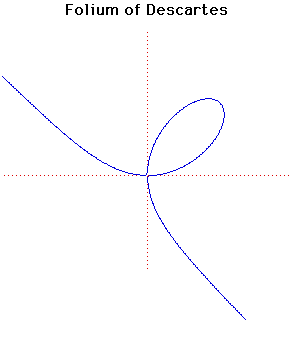# Curves

### Folium of DescartesCartesian equation:
$x^{3} + y^{3} = 3axy$
or parametrically:
$x = 3at/(1 + t^{3}), y = 3at^{2}/(1 + t^{3})$

### Description

This folium was first discussed by Descartes in 1638 but, although he found the correct shape of the curve in the positive quadrant, he believed that this leaf shape was repeated in each quadrant like the four petals of a flower.

The problem to determine the tangent to the curve was proposed to Roberval who also wrongly believed the curve had the form of a jasmine flower. His name of fleur de jasminwas later changed.

The curve is sometimes known as the noeud de ruban.

The folium has an asymptote $x + y + a = 0$.

The equation of the tangent at the point with $t = p$ is
$p(p^{3} - 2)x + (1 - 2p^{3})y + 3ap^{2} = 0$.
The curve passes through the origin at $t = 0$ and approaches the origin a second time as $t$ goes to infinity.

### Associated Curves

Definitions of the Associated curves